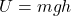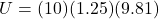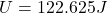## If Maria lifts a mass of 10kg into a table of 1.25m high. How much gpe has the mass gained

Question

If Maria lifts a mass of 10kg into a table of 1.25m high. How much gpe has the mass gained

in progress 0
6 months 2021-08-13T22:56:57+00:00 1 Answers 26 views 0

## Answers ( )

gravitational potential energy of 10 kg mass is 122.625 J

Explanation:

Gravitational Potential energy gain is given ashere we know that

m = 10 kg

h = 1.25 m

now from above formula we haveSo gravitational potential energy of 10 kg mass is 122.625 J